Rolle's and Mean Value Theorem

Chapter 5 Class 12 Continuity and Differentiability
Serial order wise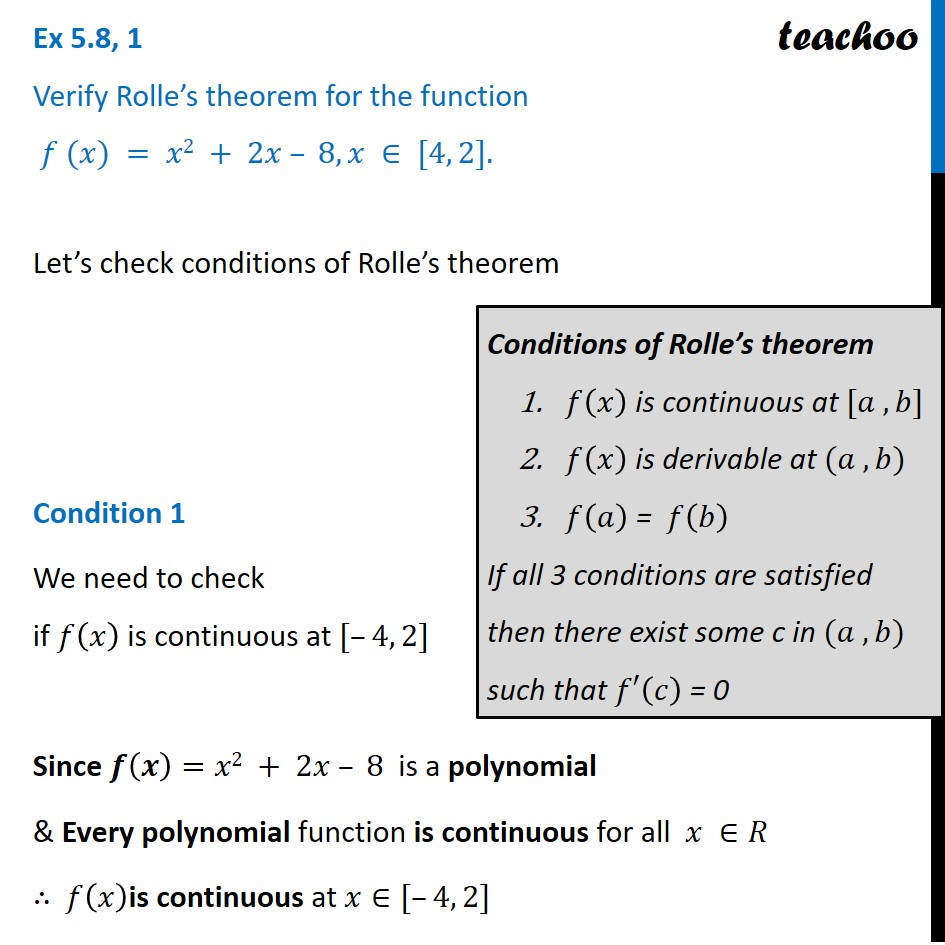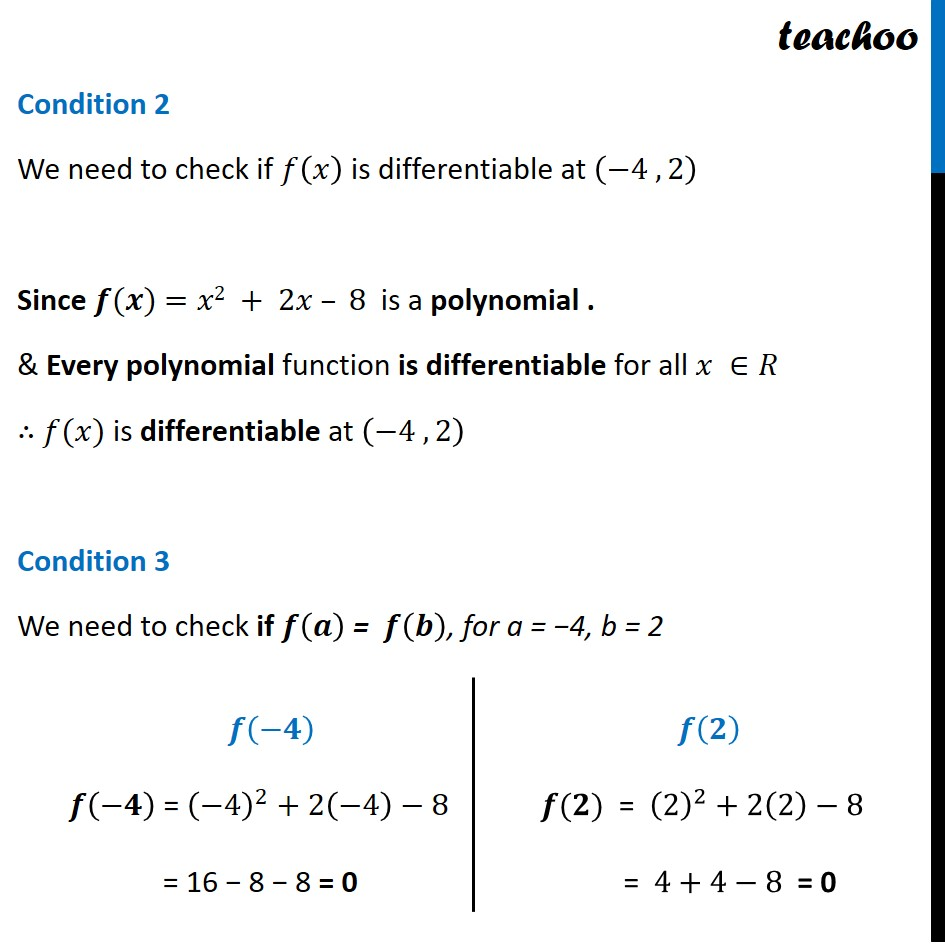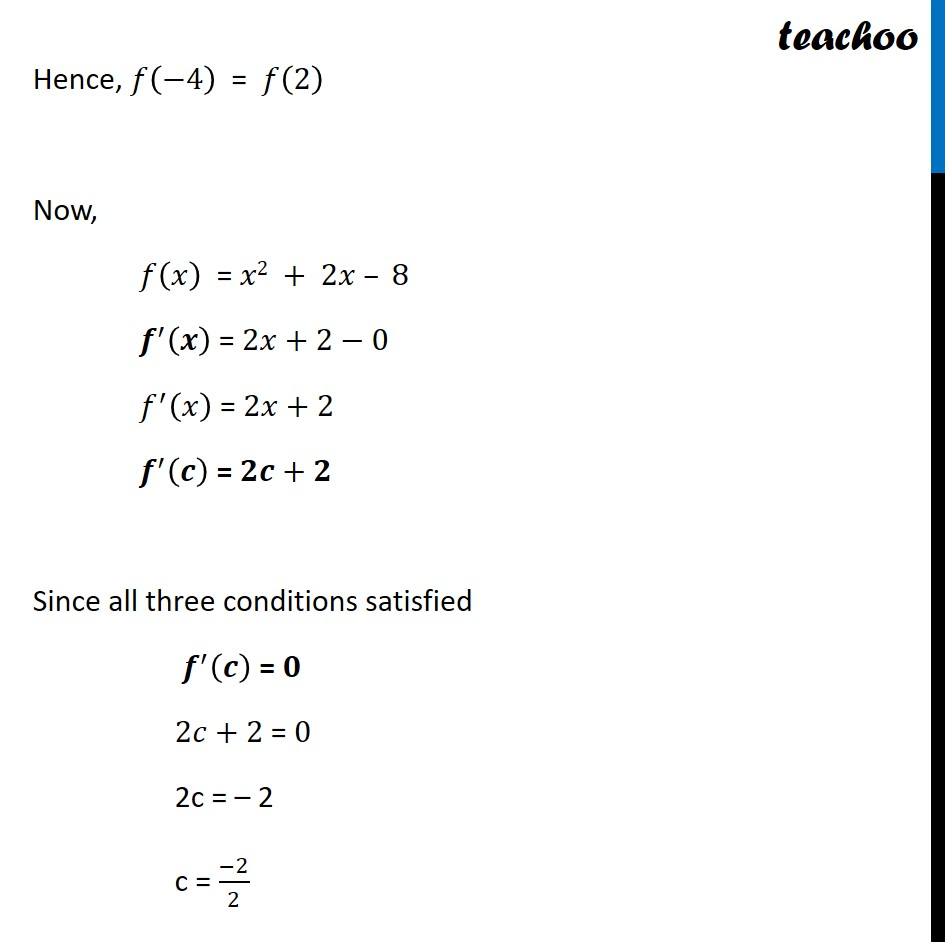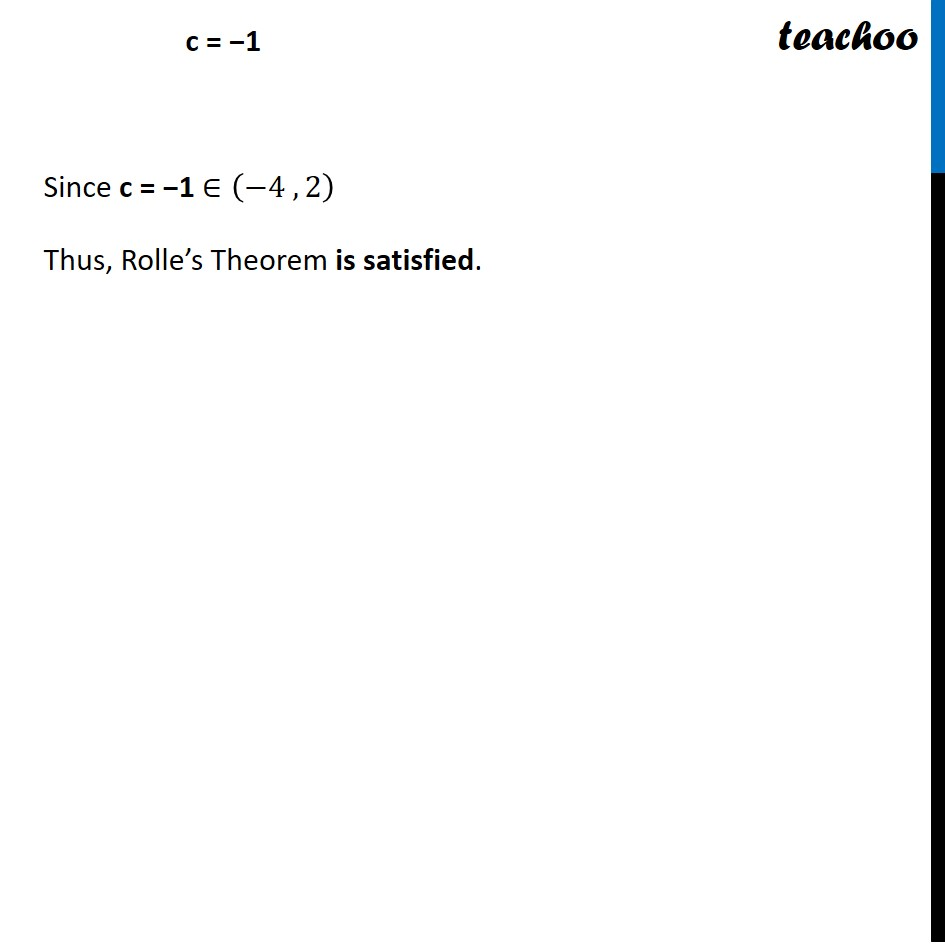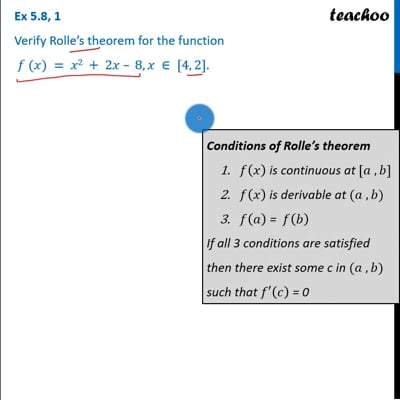This video is only available for Teachoo black usersThis video is only available for Teachoo black users

Learn in your speed, with individual attention - Teachoo Maths 1-on-1 Class

### Transcript

Question 1 Verify Rolle’s theorem for the function 𝑓 (𝑥) = 𝑥2 + 2𝑥 – 8, 𝑥 ∈ [4, 2].Let’s check conditions of Rolle’s theorem Condition 1 We need to check if 𝑓(𝑥) is continuous at [–4, 2] Since 𝒇(𝒙)=𝑥2 + 2𝑥 – 8 is a polynomial & Every polynomial function is continuous for all 𝑥 ∈𝑅 ∴ 𝑓(𝑥)is continuous at 𝑥∈[–4, 2] Conditions of Rolle’s theorem 𝑓(𝑥) is continuous at [𝑎 , 𝑏] 𝑓(𝑥) is derivable at (𝑎 , 𝑏) 𝑓(𝑎) = 𝑓(𝑏) If all 3 conditions are satisfied then there exist some c in (𝑎 , 𝑏) such that 𝑓′(𝑐) = 0 Condition 2 We need to check if 𝑓(𝑥) is differentiable at (−4 , 2) Since 𝒇(𝒙) =𝑥2 + 2𝑥 – 8 is a polynomial . & Every polynomial function is differentiable for all 𝑥 ∈𝑅 ∴ 𝑓(𝑥) is differentiable at (−4 , 2) Condition 3 We need to check if 𝒇(𝒂) = 𝒇(𝒃), for a = −4, b = 2 𝒇(−𝟒) 𝒇(−𝟒) = (−4)^2+2(−4)−8 = 16 − 8 − 8 = 0 𝒇(𝟐) 𝒇(𝟐)" = " (2)^2+2(2)−8" " "= " 4+4−8" = 0" Hence, 𝑓(−4) = 𝑓(2) Now, 𝑓(𝑥) = 𝑥2 + 2𝑥 – 8" " 𝒇^′ (𝒙) = 2𝑥+2−0 𝑓^′ (𝑥) = 2𝑥+2 𝒇^′ (𝒄) = 𝟐𝒄+𝟐 Since all three conditions satisfied 𝒇^′ (𝒄) = 𝟎 2𝑐+2 = 0 2c = – 2 c = (−2)/2 c = −1 Since c = −1 ∈(−4 , 2) Thus, Rolle’s Theorem is satisfied.